# 数据挖掘入门系列教程（二点五）之K-近邻算法和距离度量介绍

2019/04/10 10:10

## 数据挖掘入门系列教程（二点五）之K-近邻算法和距离度量介绍

### 简介

K-近邻算法，又名最近邻居算法，其英文缩写是KNN（k-nearest neighbors）。是一种用于分类和回归的非参数统计方法。其可能是标准数据挖掘算法中最为直观的一种。在这篇博客中，主要讨论knn用于分类的情况。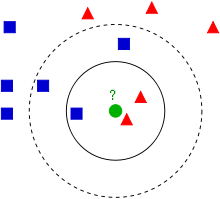• K的取值
• 距离的计算

K的取值很简单理解，因为他决定着我们需要考虑多少个”邻居“，而这个就会影响我们的模型的准确度！OK，接下来让我们来讲一讲距离度量

### 距离度量

#### 欧式距离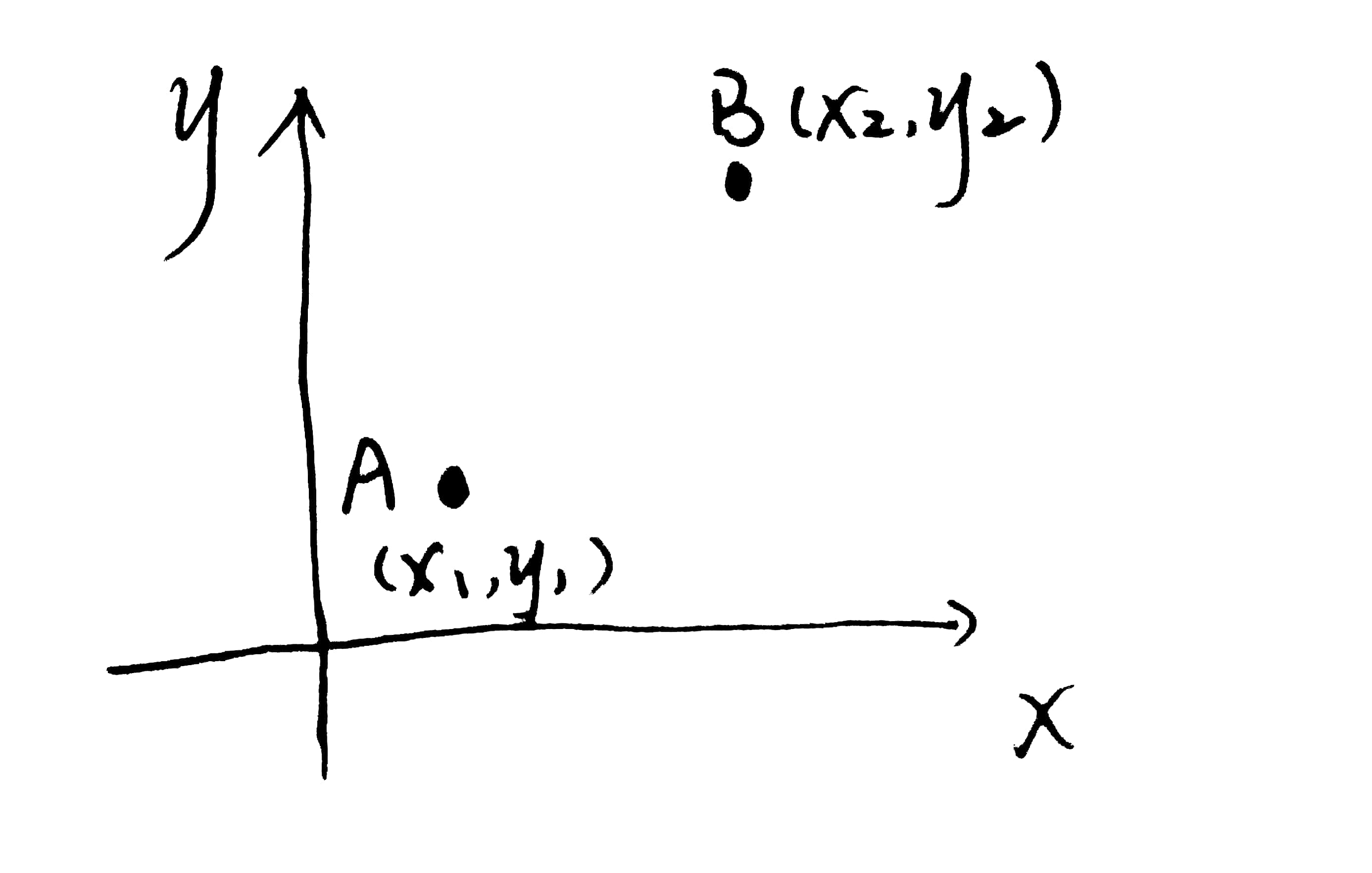emm，这个很简单，初中的时候就学过了。

$d_{AB} = \sqrt{(x_2-x_1)^2+(y_2 - y_1)^2}$

$d(x,y)=\sqrt{\Sigma_{k=1}^n (A_k-B_k)^2}\ A_k 代表A点在k轴的位置$

$d(x,y)=\sqrt{\Sigma_{k=1}^n (\dfrac{A_k-B_k}{s_k})^2}\ A_k 代表A点在k轴的位置$

#### 曼哈顿距离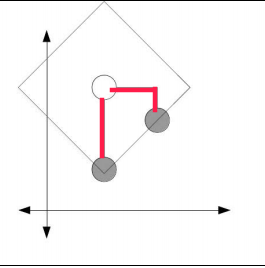$d(x,y)=\Sigma_{k=1}^n \left|A_k-B_k\right| \ k代表的是k维坐标系，A_k 代表A点在k轴的位置$

#### 余弦距离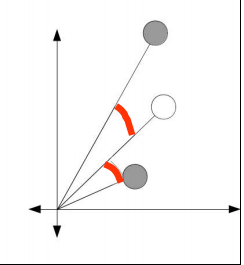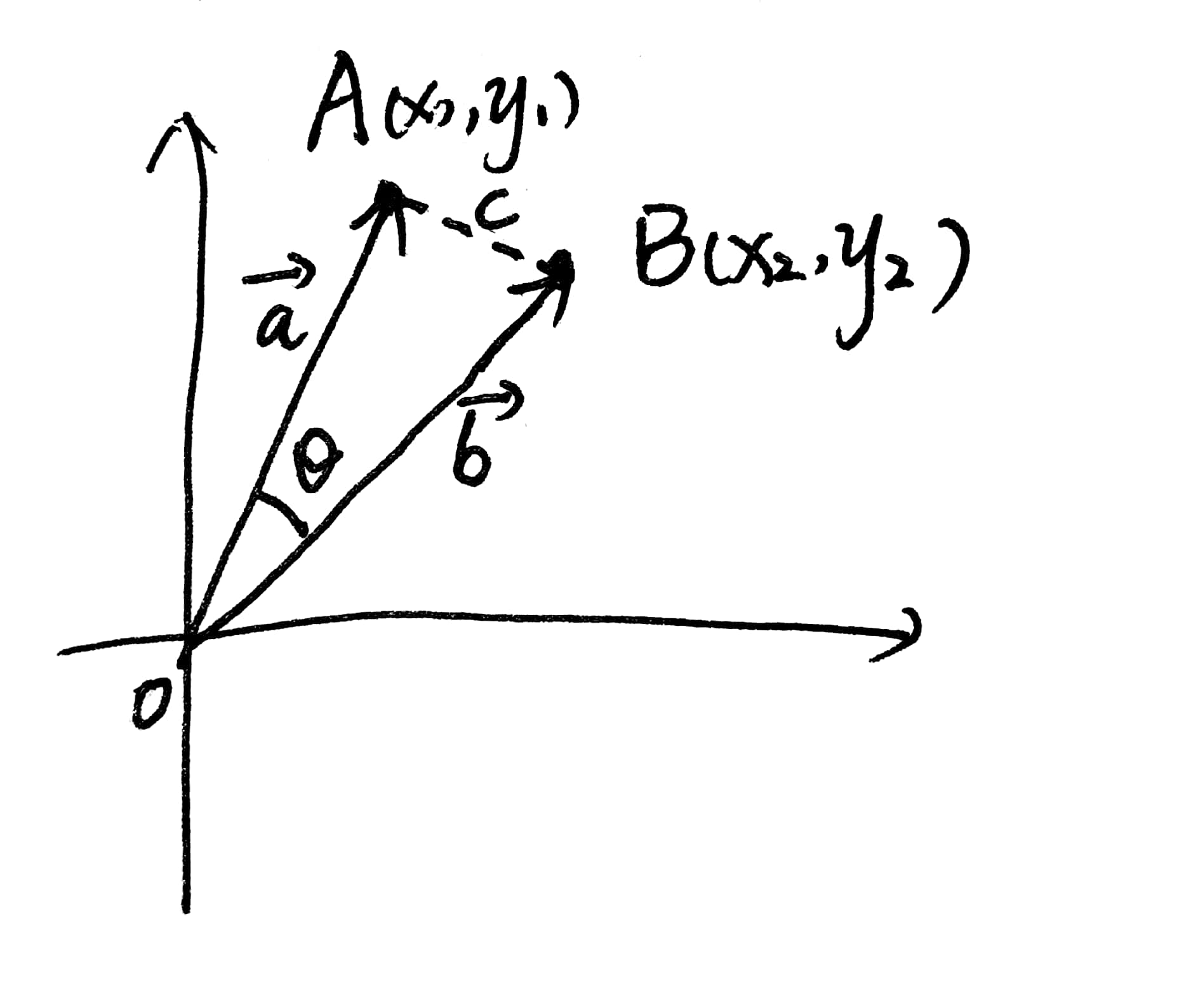$\because \overrightarrow {a} \cdot \overrightarrow{b} = |a|\times|b|\times cos\theta \ \therefore cos\theta = \frac{\overrightarrow {a} \cdot \overrightarrow{b}}{|a|\times |b|}= \dfrac{\Sigma_{k=1}^n a_k b_k}{\sqrt{\Sigma_{k=1}^n a_k^2} \sqrt{\Sigma_{k=1}^n b_k^2}}$

0
0 收藏

### 作者的其它热门文章0 评论
0 收藏
0